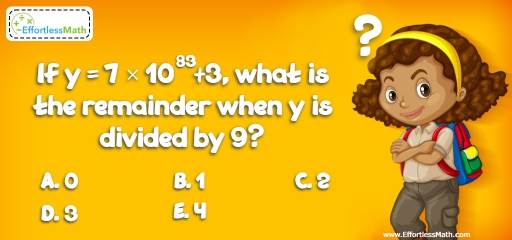# Number Properties Puzzle – Challenge 21

Math challenges are perfect learning activities to help kids learn and have fun too. Let's see who can solve this one!## Challenge:

If $$y = 7 × 10^{83}+3$$, what is the remainder when y is divided by 9?

A- 0

B- 1

C- 2

D- 3

E- 4

### The Absolute Best Book to challenge your Smart Student!

Divisibility rules for a divisor of 9:
A number is divisible by 9 if the sum of the digits is divisible by 9. For example 126 is divisible by 9, since, 1 + 2 + 6 = 9 is divisible by 9.
$$7 × 10^{83}+3 = 7, 000,…, 000 + 3 = 7,000 ,…,003$$
The sum of the digits is 10.

### What people say about "Number Properties Puzzle – Challenge 21 - Effortless Math: We Help Students Learn to LOVE Mathematics"?

No one replied yet.

X
30% OFF

Limited time only!

Save Over 30%

SAVE $5 It was$16.99 now it is \$11.99